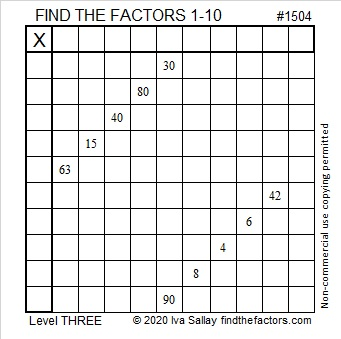# 1504 and Level 3

Contents

### Today’s Puzzle:

Since this is a level 3 puzzle the clues are given in a logical order from top to bottom. Write the factors 1 to 10 in the first column and again in the top row.

Usually, you only have to consider the previous clues when finding the factors in a level 3 puzzle, but when you consider if 4 = 2 × 2 or 1 × 4, you will also have to look at a clue below it. You can do this!### Factors of 1504:

• 1504 is a composite number.
• Prime factorization: 1504 = 2 × 2 × 2 × 2 × 2 × 47, which can be written 1504 = 2⁵ × 47.
• 1504 has at least one exponent greater than 1 in its prime factorization so √1504 can be simplified. Taking the factor pair from the factor pair table below with the largest square number factor, we get √1504 = (√16)(√94) = 4√94.
• The exponents in the prime factorization are 5 and 1. Adding one to each exponent and multiplying we get (5 + 1)(1 + 1) = 6 × 2 = 12. Therefore 1504 has exactly 12 factors.
• The factors of 1504 are outlined with their factor pair partners in the graphic below.### More about the Number 1504:

1504 is the difference of two squares in four different ways:
377² – 375² = 1504
190² – 186² = 1504
98² – 90² = 1504
55² – 39² = 1504

This site uses Akismet to reduce spam. Learn how your comment data is processed.